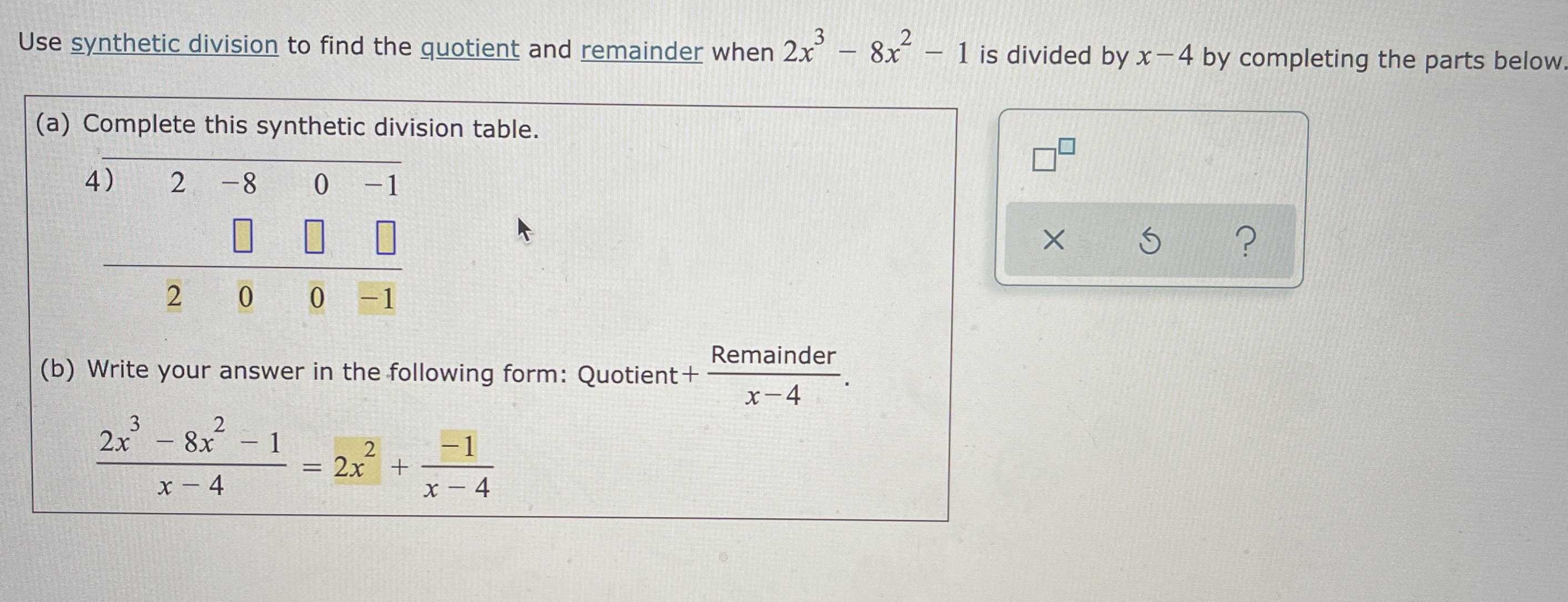### ¿Todavía tienes preguntas de matemáticas?

Pregunte a nuestros tutores expertos
Algebra
PreguntaUse synthetic division to find the quotient and remainder when $$2 x ^ { 3 } - 8 x ^ { 2 } - 1$$ is divided by $$x - 4$$ by completing the parts below.

(a) Complete this synthetic division table.

(b) Write your answer in the following form: Quotient $$\frac{Remainder}{x- 4}$$

$$\frac { 2 x ^ { 3 } - 8 x ^ { 2 } - 1 } { x - 4 } = 2 x ^ { 2 } + \frac { - 1 } { x - 4 }$$